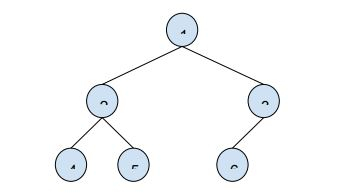# Find distance between two nodes of a Binary Tree in C++ Program

In this problem, we are given a binary tree and two nodes. Our task is to create a program to Find distance between two nodes of a Binary Tree.

## Problem Description

We need to find the distance between two nodes which is the minimum number of edges that will be traversed when we go from one node to another node.

Let’s take an example to understand the problem,

Input: binary treeNode1 = 3 ,Node2 = 5

Output: 3

## Explanation

The path from node 3 to node 5 is 3 -> 1 -> 2 -> 5. There are 3 edges traversed that make distance 3.

## Solution Approach

A simple solution to the problem is using the lowest common ancestor node for the given nodes and then apply the below formula,

distance(node1, node2) = distance(root, node1) + distance(root, node2) + distance(root, LCA)

## Example

Live Demo

#include <iostream>
using namespace std;
struct Node{
struct Node *left, *right;
int key;
};
Node* insertNode(int key){
Node *temp = new Node;
temp->key = key;
temp->left = temp->right = NULL;
return temp;
}
int calcNodeLevel(Node *root, int val, int level) {
if (root == NULL)
return -1;
if (root->key == val)
return level;
int lvl = calcNodeLevel(root->left, val, level+1);
return (lvl != -1)? lvl : calcNodeLevel(root->right, val, level+1);
}
Node *findDistanceRec(Node* root, int node1, int node2, int &dist1, int &dist2, int &dist, int lvl){
if (root == NULL) return NULL;
if (root->key == node1){
dist1 = lvl;
return root;
}
if (root->key == node2){
dist2 = lvl;
return root;
}
Node *leftLCA = findDistanceRec(root->left, node1, node2, dist1,dist2, dist, lvl+1);
Node *rightLCA = findDistanceRec(root->right, node1, node2, dist1,dist2, dist, lvl+1);
if (leftLCA && rightLCA){
dist = dist1 + dist2 - 2*lvl;
return root;
}
return (leftLCA != NULL)? leftLCA: rightLCA;
}
int CalcNodeDistance(Node *root, int node1, int node2) {
int dist1 = -1, dist2 = -1, dist;
Node *lca = findDistanceRec(root, node1, node2, dist1, dist2, dist, 1);
if (dist1 != -1 && dist2 != -1)
return dist;
if (dist1 != -1){
dist = calcNodeLevel(lca, node2, 0);
return dist;
}
if (dist2 != -1){
dist = calcNodeLevel(lca, node1, 0);
return dist;
}
return -1;
}
int main(){
Node * root = insertNode(1);
root->left = insertNode(2);
root->right = insertNode(3);
root->left->left = insertNode(4);
root->left->right = insertNode(5);
root->right->left = insertNode(6);
cout<<"Distance between node with value 5 and node with value 3 is"<<CalcNodeDistance(root, 3, 5);
return 0;
}

## Output

Distance between node with value 5 and node with value 3 is 3